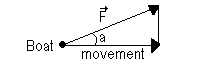## Thursday, August 28, 2008

### Work: A force on the move

It was a difficult decision, but I thought it would be better to see "work" before pressure, although I find it more difficult. But let's give it a go.

Work in physics isn't the same as work as we know it. In physics, we say work is done by a force on an object only if:
• The object displaces.
• the direction of the displacement isn't at right angles with the direction of the force.
We define this 'work' as the product of the force acting on the object and the distance through which the object moves. Or

W = F. d(x)

If we insert the units Force and Distance, we get the unit of Work, the Joule:

J = N . m

Sometimes, the direction of the force isn't the same as the direction of the movement. For example, if a boat is pulled by boatman with a rope:Here, we have a pulling force, F, which pulls in the movement direction. If we want to determine the part of the force that moves the boat, we have to project the force on the direction of the movement. If we do so, we get Fm = F . cos(a). So, the work done by the force F, with regard only to the direction of the movement, is:

W = Fm . d(x) => W = F . cos(a) . d(x)

These are the basics to work. It's probably verry abstract, but it will become clearer in the next posts about work to move objects etc.

Technorati labels: ,